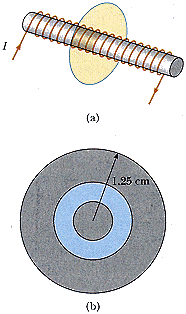# Magnetic Flux from a solenoid

Slimmy

## Homework Statement

A solenoid 2.50 cm in diameter and 26.0 cm long has 285 turns and carries 12.0 A.(a) Calculate the flux through the surface of a disk of radius 5.00 cm that is positioned perpendicular to and centered on the axis of the solenoid, as in Figure P30.36a.
(b) Figure P30.36b shows an enlarged end view of the same solenoid. Calculate the flux through the blue area, which is defined by an annulus that has an inner radius of 0.400 cm and outer radius of 0.800 cm.

## Homework Equations

Unless it wants me to use the law of Biot-Savart...I believe for a solenoid, the magnetic field is:
B=mu_0(n)L
and
Magnetic Flux =
[Surface integral](B (dot) dA)

## The Attempt at a Solution

Well, for part (a) my book states for a very long solenoid, there is no magnetic field outside of it, but I believe that is for an infinte solenoid? But in any case, I found the magnetic field assuming it was a long solenoid, then once I did that, the only part of the circle that would be in a magnetic field would be the part that is in the solenoid, so the radius is 1.25cm for the area in the magnetic flux equation, but this outlook is incorrect. For part (b)..I was too discouraged to work towards it.

Last edited by a moderator:

## Answers and Replies

nickjer
Could you please show your work for (a) so we can see where you went wrong, thanks.

Slimmy
Oh, the reason I did not show my work, is because I believed that my whole concept was incorrect...I am allowed to assume this as a very long Solenoid? One second, let me get my work real quick.

Alright, so I have the equation...

B=mu_0*n*I*1/L
and I find the magnetic field to be
.01653 T

Then I find the area that is being affected from the solenoid, and that is technically the diameter of the solenoid, so
diameter=2.5E-2 M